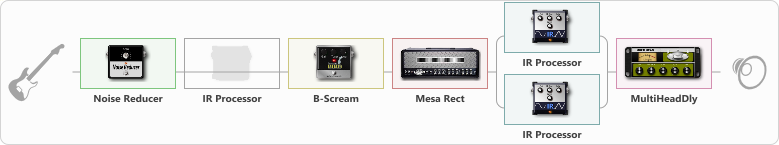# Mesa

Discussion in 'ToneLib-GFX presets' started by carlinos3, Jul 16, 2022.

1. Mesa

Preset name: Am I Evil?

Effects chain:Effect: "Noise Reducer" (Dynamics / Filter), active - "yes"
{
"Sens" = 100
"Mode" = Soft
}

Effect: "IR Processor" (Cabinets), active - "no"
{
"IR" = ditspre_dc
"Low Cut (Hz)" = 0
"Hi Cut (kHz)" = 20.0
"Mix" = 41
"Level (dB)" = 8
}

Effect: "B-Scream" (Overdrive / Distortion), active - "yes"
{
"Drive" = 67
"Tone" = 76
"Level" = 60
}

Effect: "Mesa Rect" (Amp simulators), active - "yes"
{
"Gain" = 100
"Bass" = 51
"Middle" = 73
"Treble" = 74
"Presence" = 91
"Master" = 68
"Level (dB)" = 0
}

Effect: "Splitter" (Dynamics / Filter)
{
"A-Bypass" = Off
"A-Pan" = 0
"A-Level" = 55
"B-Bypass" = Off
"B-Pan" = 0
"B-Level" = 56
"Width" = 0

'A' branch:
{

Effect: "IR Processor" (Cabinets), active - "yes"
{
"IR" = imp-feb-3_dc
"Low Cut (Hz)" = 0
"Hi Cut (kHz)" = 20.0
"Mix" = 100
"Level (dB)" = 4
}
}
'B' branch:
{

Effect: "IR Processor" (Cabinets), active - "yes"
{
"IR" = Lucia-111_dc
"Low Cut (Hz)" = 0
"Hi Cut (kHz)" = 20.0
"Mix" = 100
"Level (dB)" = 7
}
}
}

Effect: "MultiHeadDly" (Delay), active - "yes"
{
"Time" = 345
"Feedback" = 30
"Tone" = 58
"MODE" = Rhythm X-0-X-X
"Mix" = 49
}

Note: You will need to download and install the ToneLib-GFX software to use the preset.

#### Attached Files:

• ###### Mesa.tlgfx
File size:
86.5 KB
Views:
1,772
IpatiyPotiy and TheAragones like this.